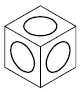# ELLIPSE (Command)

Creates an ellipse or an elliptical arc.

Find

The first two points of the ellipse determine the location and length of the first axis. The third point determines the distance between the center of the ellipse and the end point of the second axis.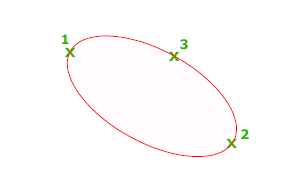The following prompts are displayed.

### Axis Endpoint

Defines the first axis by its two endpoints. The angle of the first axis determines the angle of the ellipse. The first axis can define either the major or the minor axis of the ellipse.

Distance to Other Axis

Defines the second axis using the distance from the midpoint of the first axis to the endpoint of the second axis (3).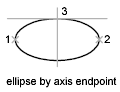Rotation

Creates the ellipse by appearing to rotate a circle about the first axis.

Move the crosshairs around the center of the ellipse and click. If you enter a value, the higher the value, the greater the eccentricity of the ellipse. Entering 0 defines a circular ellipse.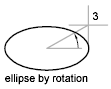### Arc

Creates an elliptical arc.

The angle of the first axis determines the angle of the elliptical arc. The first axis can define either the major or the minor axis depending on its size.

The first two points of the elliptical arc determine the location and length of the first axis. The third point determines the distance between the center of the elliptical arc and the endpoint of the second axis. The fourth and fifth points are the start and end angles.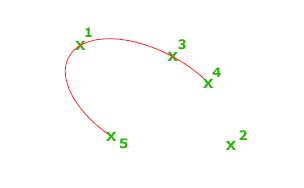Axis Endpoint

Defines the start point of the first axis.

Rotation

Defines the major to minor axis ratio of the ellipse by rotating a circle about the first axis. The higher the value from 0 through 89.4 degrees, the greater the ratio of minor to major axis. Values between 89.4 degrees and 90.6 degrees are invalid because the ellipse would otherwise appear as a straight line. Multiples of these angle values result in a mirrored effect every 90 degrees.

Start Angle

Defines the first endpoint of the elliptical arc. The Start Angle option also changes Parameter mode to Angle mode. The mode controls how the ellipse is calculated.

Parameter (specialized option)

Requires angular input, but creates the elliptical arc using the following parametric vector equation for the angle of each endpoint:

p(angle) = c + a * cos(angle) + b * sin(angle)

where c is the center of the ellipse and a and b are the negative lengths of its major and minor axes, respectively.

• End Parameter: Defines the end angle of the elliptical arc by using a parametric vector equation. The Start Parameter option toggles from Angle mode to Parameter mode. The mode controls how the ellipse is calculated.
• Angle: Defines the end angle of the elliptical arc. The Angle option toggles from Parameter mode to Angle mode. The mode controls how the ellipse is calculated.
• Included Angle: Defines an included angle beginning at the start angle.

### Center

Creates an ellipse using a center point, the endpoint of the first axis, and the length of the second axis. You can specify the distances by clicking a location at the desired distance or by entering a value for the length.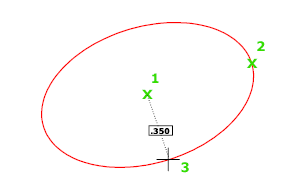Distance to Other Axis

Defines the second axis as the distance from the center of the ellipse, or midpoint of the first axis, to the point you specify.

Rotation

Creates the ellipse by appearing to rotate a circle about the first axis.

Move the crosshairs around the center of the ellipse and click. If you enter a value, the higher the value, the greater the eccentricity of the ellipse. Entering 0 defines a circle.

### Isocircle

Creates an isometric circle in the current isometric drawing plane.

Note: The Isocircle option is available only when ISODRAFT is set to an isoplane, or the Style option of SNAP is set to Isometric.